# Binary Tree | Binary Tree Properties

## Binary Tree-

Before you go through this article, make sure that you gone through the previous article on Binary Trees.

We have discussed-

• Binary tree is a special tree data structure.
• In a binary tree, each node can have at most 2 children.
• There are following types of binary trees-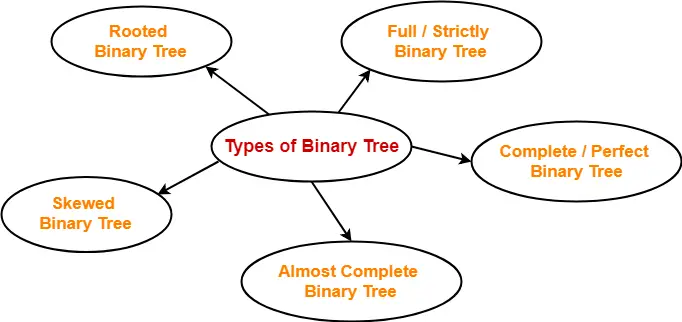## Binary Tree Properties-

Important properties of binary trees are-

## Property-01:

 Minimum number of nodes in a binary tree of height H = H + 1

### Example-

To construct a binary tree of height = 4, we need at least 4 + 1 = 5 nodes.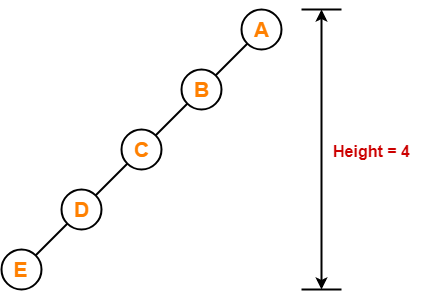## Property-02:

 Maximum number of nodes in a binary tree of height H = 2H+1 – 1

### Example-

Maximum number of nodes in a binary tree of height 3

= 23+1 – 1

= 16 – 1

= 15 nodes

Thus, in a binary tree of height = 3, maximum number of nodes that can be inserted = 15.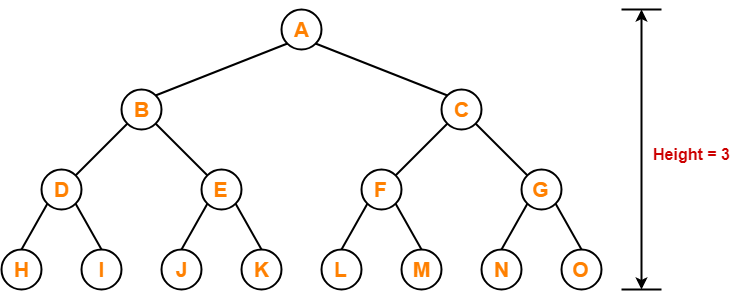We can not insert more number of nodes in this binary tree.

## Property-03:

 Total Number of leaf nodes in a Binary Tree = Total Number of nodes with 2 children + 1

### Example-

Consider the following binary tree-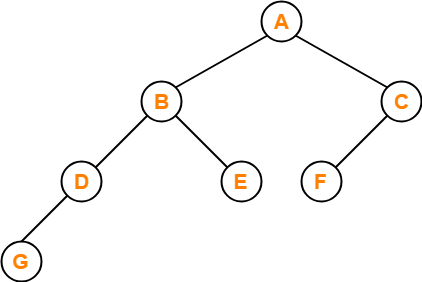Here,

• Number of leaf nodes = 3
• Number of nodes with 2 children = 2

Clearly, number of leaf nodes is one greater than number of nodes with 2 children.

This verifies the above relation.

## NOTE

It is interesting to note that-

Number of leaf nodes in any binary tree depends only on the number of nodes with 2 children.

## Property-04:

 Maximum number of nodes at any level ‘L’ in a binary tree = 2L

### Example-

Maximum number of nodes at level-2 in a binary tree

= 22

= 4

Thus, in a binary tree, maximum number of nodes that can be present at level-2 = 4.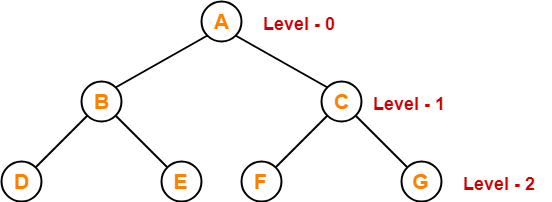To gain better understanding about Binary Tree Properties,

Watch this Video Lecture

## Problem-01:

A binary tree T has n leaf nodes. The number of nodes of degree-2 in T is ______?

1. log2n
2. n-1
3. n
4. 2n

## Solution-

Using property-3, we have-

Number of degree-2 nodes

= Number of leaf nodes – 1

= n – 1

Thus, Option (B) is correct.

## Problem-02:

In a binary tree, for every node the difference between the number of nodes in the left and right subtrees is at most 2. If the height of the tree is h > 0, then the minimum number of nodes in the tree is ______?

1. 2h-1
2. 2h-1 + 1
3. 2h – 1
4. 2h

## Solution-

Let us assume any random value of h. Let h = 3.

Then the given options reduce to-

1. 4
2. 5
3. 7
4. 8

Now, consider the following binary tree with height h = 3-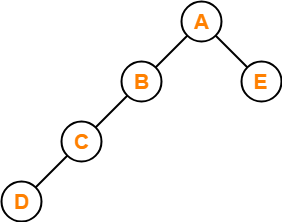• This binary tree satisfies the question constraints.
• It is constructed using minimum number of nodes.

Thus, Option (B) is correct.

## Problem-03:

In a binary tree, the number of internal nodes of degree-1 is 5 and the number of internal nodes of degree-2 is 10. The number of leaf nodes in the binary tree is ______?

1. 10
2. 11
3. 12
4. 15

## Solution-

Using property-3, we have-

Number of leaf nodes in a binary tree

= Number of degree-2 nodes + 1

= 10 + 1

= 11

Thus, Option (B) is correct.

## Problem-04:

The height of a binary tree is the maximum number of edges in any root to leaf path. The maximum number of nodes in a binary tree of height h is ______?

1. 2h
2. 2h-1 – 1
3. 2h+1 – 1
4. 2h+1

## Solution-

Using property-2, Option (C) is correct.

## Problem-05:

A binary tree T has 20 leaves. The number of nodes in T having 2 children is ______?

## Solution-

Using property-3, correct answer is 19.

To watch video solutions and practice more problems,

Watch this Video LectureNext Article- Binary Tree Traversal

Get more notes and other study material of Data Structures.

Watch video lectures by visiting our YouTube channel LearnVidFun.

SummaryArticle Name
Binary Tree | Binary Tree Properties
Description
Binary tree is a special tree data structure. There are various types of binary trees. Binary Tree Properties are given. If height of binary tree = H then, minimum number of nodes in binary tree H+1.
Author
Publisher Name
Gate Vidyalay
Publisher Logo